Courses

# Test: Conditions For A Rigid-Body Equilibrium

## 30 Questions MCQ Test Engineering Mechanics | Test: Conditions For A Rigid-Body Equilibrium

Description
This mock test of Test: Conditions For A Rigid-Body Equilibrium for Mechanical Engineering helps you for every Mechanical Engineering entrance exam. This contains 30 Multiple Choice Questions for Mechanical Engineering Test: Conditions For A Rigid-Body Equilibrium (mcq) to study with solutions a complete question bank. The solved questions answers in this Test: Conditions For A Rigid-Body Equilibrium quiz give you a good mix of easy questions and tough questions. Mechanical Engineering students definitely take this Test: Conditions For A Rigid-Body Equilibrium exercise for a better result in the exam. You can find other Test: Conditions For A Rigid-Body Equilibrium extra questions, long questions & short questions for Mechanical Engineering on EduRev as well by searching above.
QUESTION: 1

### The main condition for the rigid body is that the distance between various particles of the body does change.

Solution:

The main condition for the rigid body is that the distance between various particles of the body does not change. If the distance does change the body is not to be called as rigid. Thus the fix distance of the particles is very much required for the equilibrium of the rigid body.

QUESTION: 2

### The shown here has a mass of 100kg. What is missing here in the diagram?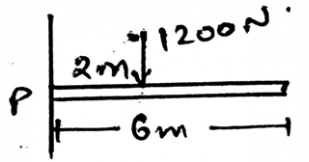Solution:

The main condition for the rigid body is that the distance between various particles of the body does not change. If the distance does change the body is not to be called as rigid. Thus the fix distance of the particles is very much required for the equilibrium of the rigid body.

QUESTION: 3

### What does the Newton’s third law states?

Solution:

The requirement of the third law is important in the equilibrium of the body. Specially the rigid bodies. The rigid body particles are in the equilibrium and are thus facing the forces and to be in the equilibrium they also react and apply the opposite force and thus the third law of newton.

QUESTION: 4

Determine the horizontal components of the reaction on the beam caused by the pin at P. The force 60N is multiplied by 10 and then is applied.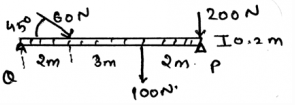Solution:

The summation of the forces needs to be zero. So does the summation of the moments need to zero. But talking about the angles, they not needed to zero. But the forces which are acting at particular angles, must needed to be equal to zero. The basic need of the forces to make the body in equilibrium.

QUESTION: 5

For equilibrium the net force acting on the body is zero.

Solution:

The equilibrium is only attained if the net force on the body tends to be equal to zero. Thus the forces cancels out. If this happens there is no motion of the body along any direction and hence the body is said to be in equilibrium. The body here is a rigid body.

QUESTION: 6

For equilibrium the net moment acting on the body by various forces is zero.

Solution:

The equilibrium is only attained if the net moment on the body tends to be equal to zero. Thus the moments caused by different forces cancels out. If this happens there is no motion of the body along any direction and hence the body is said to be in equilibrium. The body here is a rigid body.

QUESTION: 7

Determine the vertical components of the reaction on the beam caused by the pin at Q. The force 60N is multiplied by 10 and then is applied.

Solution:

The summation of the forces needs to be zero. So does the summation of the moments need to zero. But talking about the angles, they not needed to zero. But the forces which are acting at particular angles, must needed to be equal to zero. The basic need of the forces to make the body in equilibrium.

QUESTION: 8

The net moment of the body is zero that means the distance between the force and the rotational axis is zero.

Solution:

The net moment of the body is zero that doesn’t means that the distance between the force and the rotational axis is zero. This means moments caused by different forces cancels out. If this happens there is no motion of the body along any direction and hence the body is said to be in equilibrium.

QUESTION: 9

Determine the horizontal components of the reaction on the beam caused by the roller at P.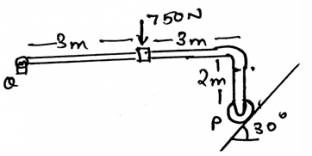Solution:

The summation of the forces needs to be zero. So does the summation of the moments need to zero. But talking about the angles, they not needed to zero. But the forces which are acting at particular angles, must needed to be equal to zero. The basic need of the forces to make the body in equilibrium.

QUESTION: 10

The net force of the body is zero that means the force are not being applied to the body at all and hence the body is in equilibrium.

Solution:

The net force of the body is zero that doesn’t means that the force are not being applied to the body at all and hence the body is in equilibrium. The equilibrium is only attained if the net force on the body tends to be equal to zero. Thus the forces cancels out. If this happens there is no motion of the body along any direction and hence the body is said to be in equilibrium.

QUESTION: 11

Which of the following is correct?

Solution:

The application of the conditions of the equilibrium of the body is valid throughout. This means that the conditions are irrespective of the dimensions. The conditions are the basic rules that defines the equilibrium of the body and thus are applicable in any dimension of the real axis.

QUESTION: 12

Determine the vertical components of the reaction on the beam caused by the roller at P.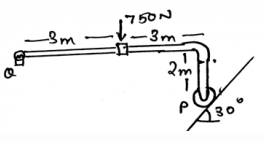Solution:

The summation of the forces needs to be zero. So does the summation of the moments need to zero. But talking about the angles, they not needed to zero. But the forces which are acting at particular angles, must needed to be equal to zero. The basic need of the forces to make the body in equilibrium.

QUESTION: 13

For the conditions of the equilibrium of the body, i.e. the rigid body only the external forces defines the equilibrium. Because the internal forces cancels out so not to be considered.

Solution:

The application of the internal forces does affect the conditions of the equilibrium of the body. Not only the external but the internal forces that are developed by the sake of external forces does develop a tending effect on the equilibrium of the body. Thus the internal forces doesn’t cancels out.

QUESTION: 14

Which of the following needs to zero for the perfect equilibrium?

Solution:

The summation of the forces needs to be zero. So does the summation of the moments need to zero. But talking about the angles, they not needed to zero. But the forces which are acting at particular angles, must needed to be equal to zero. The basic need of the forces to make the body in equilibrium.

QUESTION: 15

Determine the vertical components of the reaction on the beam caused by the pin at P. The force 60N is multiplied by 10 and then is applied.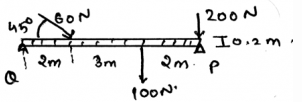Solution:

The summation of the forces needs to be zero. So does the summation of the moments need to zero. But talking about the angles, they not needed to zero. But the forces which are acting at particular angles, must needed to be equal to zero. The basic need of the forces to make the body in equilibrium.

QUESTION: 16

Free body diagrams doesn’t play any role in making the calculations on the conditions of the equilibrium of the body.

Solution:

The free body diagrams does play an important role in the formation of the conditions of the equilibrium of the rigid body. As the net forces are zero, the fbd helps us to take the measure of the same. That is to see whether the summation is really zero or not.

QUESTION: 17

The net forces of acting on the body needs to be zero. This is also applicable for the simply supported beams. This means that the support reaction are also counted in making the net force zero.

Solution:

The support reactions of the beam is also counted in the making of the forces zero. As far as the net force is concerned the support reaction does affect the conditions for the equilibrium of the body. Hence one needs to take care of the support reactions of the beam too.

QUESTION: 18

Determine the horizontal components of the reaction on the beam caused by the pin at Q.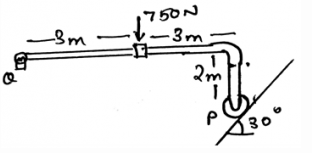Solution:

The summation of the forces needs to be zero. So does the summation of the moments need to zero. But talking about the angles, they not needed to zero. But the forces which are acting at particular angles, must needed to be equal to zero. The basic need of the forces to make the body in equilibrium.

QUESTION: 19

If the body is in equilibrium, but it having a rotational curled ray shown in the free body diagram then:

Solution:

The body having equilibrium will not rotate at any cost. Yes, the diagram may contain the rotational array showing the couple being acted over the structure. But the thing is that the forces, i.e. the other forces which are outside the dependency of this rotation will cancel out this rotation and thus the body is in equilibrium.

QUESTION: 20

If five forces are acting on the single particle and having an angle of 72˚ between each and are collinear, then:

Solution:

The net force acting on the body is zero. This means that the forces cancel out. This means that the body is in equilibrium and doesn’t need any of the external force to make itself in the equilibrium.

QUESTION: 21

Which of the following is correct?

Solution:

The support is the thing which a human decides. That can be anything. That is the support can be given in any way. The type of support also is important as different types of supports have different conditions for the equilibrium.

QUESTION: 22

What does the Newton’s second law states?

Solution:

The requirement of the second law is important in the equilibrium of the body. Specially the rigid bodies. The rigid body particles are if are in motion then this law helps in making the conditions for the equilibrium of the body. As when the body will leave its state of motion and come in rest, etc.

QUESTION: 23

Determine the vertical components of the reaction on the beam caused by the pin at Q.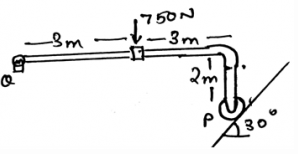Solution:

The summation of the forces needs to be zero. So does the summation of the moments need to zero. But talking about the angles, they not needed to zero. But the forces which are acting at particular angles, must needed to be equal to zero. The basic need of the forces to make the body in equilibrium.

QUESTION: 24

Which of the following is correct?

Solution:

The application of the conditions of the equilibrium of the body is valid throughout. This means that the conditions are irrespective of the types of forces. The conditions are the basic rules that defines the equilibrium of the body and thus are applicable in any type of forces of the real axis.

QUESTION: 25

Determine the vertical components of the reaction on the beam caused by the pin at Q. The force 60N is multiplied by 10 and then is applied.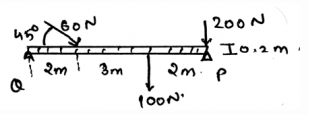Solution:

The summation of the forces needs to be zero. So does the summation of the moments need to zero. But talking about the angles, they not needed to zero. But the forces which are acting at particular angles, must needed to be equal to zero. The basic need of the forces to make the body in equilibrium.

QUESTION: 26

For the conditions of the equilibrium of the body, i.e. the rigid body only the external forces defines the equilibrium. And the support reactions only cancels out the rotation part of the body.

Solution:

The application of the support reaction forces does affect the conditions of the equilibrium of the body. Not only the external but the support reaction forces that are developed by the sake of external forces does develop a tending effect on the equilibrium of the body. Thus the support reaction forces also cancels the external forces.

QUESTION: 27

Cantilever beams are always in equilibrium, whether you form the equilibrium equations or not.

Solution:

The thing is that the formation of the conditions only verifies that the body is in equilibrium or not. Thus if the cantilever is in equilibrium then also the condition needs to be applied. It will be wrong to justify the equilibrium only on the basis of structure.

QUESTION: 28

Determine the horizontal components of the reaction on the beam caused by the pin at Q.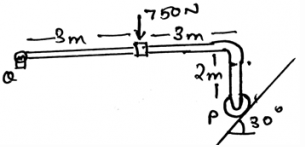Solution:

The summation of the forces needs to be zero. So does the summation of the moments need to zero. But talking about the angles, they not needed to zero. But the forces which are acting at particular angles, must needed to be equal to zero. The basic need of the forces to make the body in equilibrium.

QUESTION: 29

Determine the vertical components of the reaction on the beam caused by the pin at Q. The force 60N is multiplied by 10 and then is applied.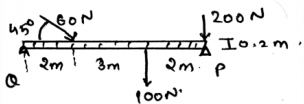Solution:

The summation of the forces needs to be zero. So does the summation of the moments need to zero. But talking about the angles, they not needed to zero. But the forces which are acting at particular angles, must needed to be equal to zero. The basic need of the forces to make the body in equilibrium.

QUESTION: 30

For the conditions of the equilibrium of the body, i.e. the rigid body only the external forces defines the equilibrium. And the support reactions only adds the rotation part of the body.

Solution:

The application of the support reaction forces does affect the conditions of the equilibrium of the body. Not only the external but the support reaction forces that are developed by the sake of external forces does develop a tending effect on the equilibrium of the body. Thus the support reaction forces also cancels the external forces.